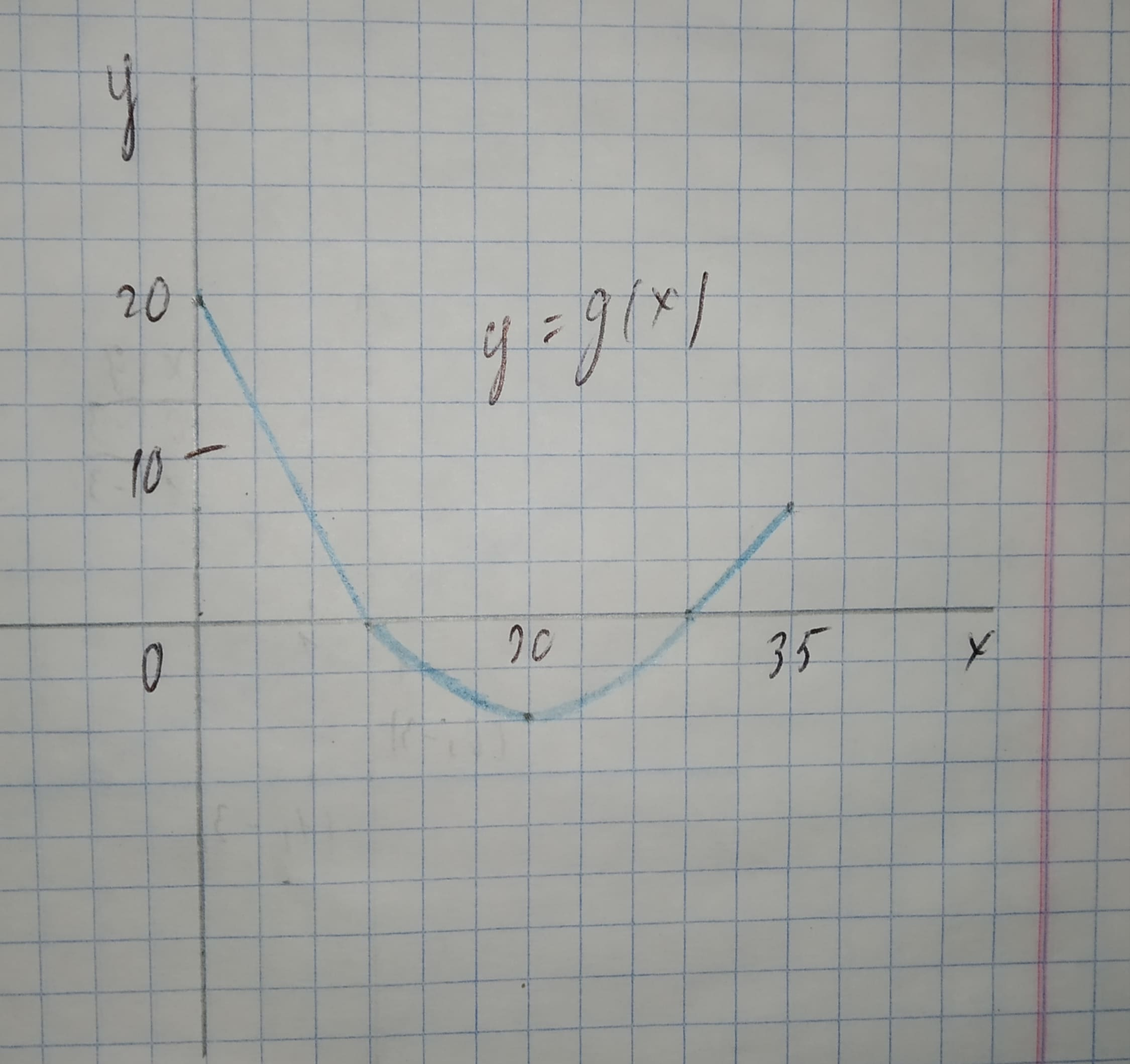# The graph of g consists of two straight lines and a semicircle. Use it to eveluate the integral.12210203861.jpgint_10^30 g(x)dxAlbarellak 2021-02-05 Answered

The graph of g consists of two straight lines and a semicircle. Use it to eveluate the integral.${\int }_{10}^{30}g\left(x\right)dx$

You can still ask an expert for help

• Questions are typically answered in as fast as 30 minutes

Solve your problem for the price of one coffee

• Math expert for every subject
• Pay only if we can solve itAniqa O'Neill

The integral ${\int }_{10}^{30}g\left(x\right)dx$ is the area between the graph of g and the horizontal x-axis on the interval $10\le x\le 30$.
We note that this area forms a semicircle with radius r = 10. Since the semicricle lies below the horizontal axis, the integral will be negative.
The area of a circle is the product of $\pi$ and the squared radius ${r}^{2}$. The area of a semicircle is half the area of a circle.
${\int }_{10}^{30}g\left(x\right)dx=-\frac{\pi {r}^{2}}{2}$
$=-\frac{\pi \left({10}^{2}\right)}{2}$
$=-\frac{100\pi }{2}$
$=-50\pi$
$\approx -157.0796$